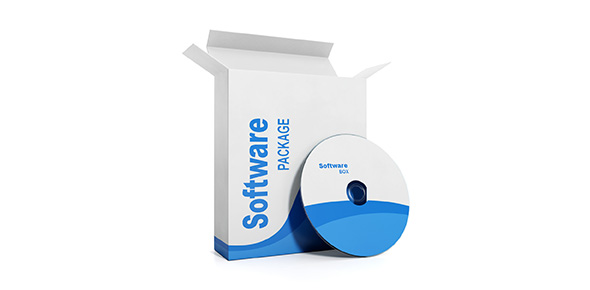# Eclipse Modeling Framework (EMF) Quiz Questions

8 Questions | Total Attempts: 64SettingsAn electro motive force is the electric energy that is transferred in a circuit or device. Having covered how to calculate the emf take the quiz below and test your new found knowledge by working out the set of questions below. Have fun answering them and not the point where you need clarifications.

• 1.
Define EMF.
• 2.
A battery with an EMF of 12V and internal resistance of 4 ohms is connected across a 20 ohm load.  What will the current be?
• A.

0.5A

• B.

0.6A

• C.

3A

• D.

1.66A

• E.

2A

• 3.
The battery with EMF of 12 V and internal resistance of 4 ohms is connected across a 20 ohm load (as above) What will the PD across the load be?
• A.

12V

• B.

10V

• C.

8V

• D.

6V

• 4.
A battery with EMF of 12V and with internal resistance of 4 ohms is connected across a 8 ohm load.  What is the PD across the load.
• A.

12V

• B.

10V

• C.

8V

• D.

6V

• 5.
What is the condition that the PD across the battery as measured by a voltmeter will actually equal the EMF of the battery?
• A.

Load is equal to the internal resistance.

• B.

• C.

• D.

Current is large.

• E.

Current is small.

• 6.
Two sets of readings are taken with a battery whose EMF is 6V.   When a 9 ohm resistor is placed across the battery, the current is 0.5A. When a 27 ohm resistor is placed across the battery, the current is reduced to 0.2A. What is the internal resistance of the battery?
• A.

1 ohm

• B.

2 ohms

• C.

3 ohms

• D.

4 ohms

• E.

5 ohms

• F.

6 ohms

• 7.
Are there any parts of this topic EMF and internal resistance you would like to review in class?  Be specific.
• 8.
Select the ones you like
• A.

Option 1

• B.

Option 2

• C.

Option 3

• D.

Option 4

Related TopicsBack to top